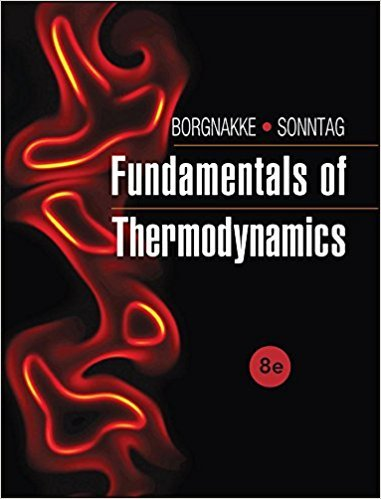×
Get Full Access to Fundamentals Of Thermodynamcs - 8 Edition - Chapter 6 - Problem 21hp
Get Full Access to Fundamentals Of Thermodynamcs - 8 Edition - Chapter 6 - Problem 21hp

×

# Assume that the heat engine in 5.34 has a high temperatureISBN: 9781118131992 58

## Solution for problem 21HP Chapter 6

Fundamentals of Thermodynamcs | 8th Edition

• Textbook Solutions
• 2901 Step-by-step solutions solved by professors and subject experts
• Get 24/7 help from StudySoup virtual teaching assistantsFundamentals of Thermodynamcs | 8th Edition

4 5 1 343 Reviews
24
1
Problem 21HP

Problem 21HP

Assume that the heat engine in Problem 5.34 has a high temperature of 1000 K and a low temperature of 400 K. What does the inequality of Clausius say about each of the four cases?

Problem 5.34

For each of the cases below, determine if the heat engine satisfies the first law (energy equation) and if it violates the second law.

a. ˙Q H = 6 kW, ˙QL = 4 kW, ˙W = 2 kW

b. ˙Q H = 6 kW, ˙QL = 0 kW, ˙W = 6 kW

c. ˙Q H = 6 kW, ˙QL = 2 kW, ˙W = 5 kW

d. ˙Q H = 6 kW, ˙QL = 6 kW, ˙W = 0 kW

Step-by-Step Solution:
Step 1 of 3

Meteorology Day #8 9/19/16 Week 5 Lecture Notes Continued Elevation: temperature us warmer at ground level than at mountain peak Latitudinal Variation: Balance is seen around latitudes 38 degrees N and 38 degrees S Poles have negative net radiation Equator has positive net radiation Wind and ocean currents redistribute energy mainly through convection Air Temperature Data: Monthly Mean: avg. of daily me

Step 2 of 3

Step 3 of 3

##### ISBN: 9781118131992

Unlock Textbook Solution